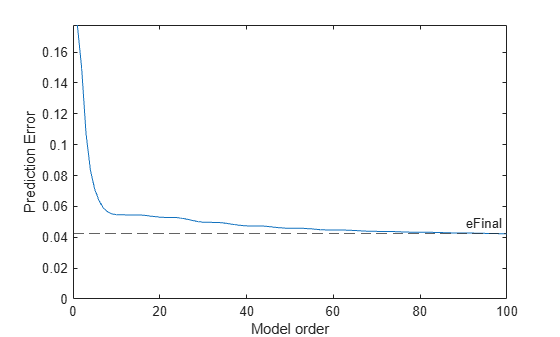# rlevinson

Reverse Levinson-Durbin recursion

## Syntax

```r = rlevinson(a,efinal) [r,u] = rlevinson(a,efinal) [r,u,k] = rlevinson(a,efinal) [r,u,k,e] = rlevinson(a,efinal) ```

## Description

The reverse Levinson-Durbin recursion implements the step-down algorithm for solving the following symmetric Toeplitz system of linear equations for r, where r = [r(1) … r(p + 1)] and r(i)* denotes the complex conjugate of r(i).

`$\left[\begin{array}{cccc}r\left(1\right)& r{\left(2\right)}^{\ast }& \cdots & r{\left(p\right)}^{\ast }\\ r\left(2\right)& r\left(1\right)& \cdots & r{\left(p-1\right)}^{\ast }\\ ⋮& \ddots & \ddots & ⋮\\ r\left(p\right)& \cdots & r\left(2\right)& r\left(1\right)\end{array}\right]\left[\begin{array}{c}a\left(2\right)\\ a\left(3\right)\\ ⋮\\ a\left(p+1\right)\end{array}\right]=\left[\begin{array}{c}-r\left(2\right)\\ -r\left(3\right)\\ ⋮\\ -r\left(p+1\right)\end{array}\right]$`

`r = rlevinson(a,efinal)` solves the above system of equations for r given vector a, where a = [1 a(2) … a(p + 1)]. In linear prediction applications, `r` represents the autocorrelation sequence of the input to the prediction error filter, where r(1) is the zero-lag element. The figure below shows the typical filter of this type, where H(z) is the optimal linear predictor, x(n) is the input signal, $\stackrel{^}{x}\left(n\right)$ is the predicted signal, and e(n) is the prediction error.Input vector a represents the polynomial coefficients of this prediction error filter in descending powers of z.

`$A\left(z\right)=1+a\left(2\right){z}^{-1}+\cdots +a\left(n+1\right){z}^{-p}$`

The filter must be minimum-phase to generate a valid autocorrelation sequence. `efinal` is the scalar prediction error power, which is equal to the variance of the prediction error signal, σ2(e).

`[r,u] = rlevinson(a,efinal)` returns upper triangular matrix U from the UDU* decomposition

`${R}^{-1}=U{E}^{-1}{U}^{\ast }$`

where

`$R=\left[\begin{array}{cccc}r\left(1\right)& r{\left(2\right)}^{\ast }& \cdots & r{\left(p\right)}^{\ast }\\ r\left(2\right)& r\left(1\right)& \cdots & r{\left(p-1\right)}^{\ast }\\ ⋮& \ddots & \ddots & ⋮\\ r\left(p\right)& \cdots & r\left(2\right)& r\left(1\right)\end{array}\right]$`

and E is a diagonal matrix with elements returned in output `e` (see below). This decomposition permits the efficient evaluation of the inverse of the autocorrelation matrix, R−1.

Output matrix `u` contains the prediction filter polynomial, `a`, from each iteration of the reverse Levinson-Durbin recursion

`$U=\left[\begin{array}{cccc}{a}_{1}{\left(1\right)}^{\ast }& {a}_{2}{\left(2\right)}^{\ast }& \cdots & {a}_{p+1}{\left(p+1\right)}^{\ast }\\ 0& {a}_{2}{\left(1\right)}^{\ast }& \ddots & {a}_{p+1}{\left(p\right)}^{\ast }\\ 0& 0& \ddots & {a}_{p+1}{\left(p-1\right)}^{\ast }\\ ⋮& \ddots & \ddots & ⋮\\ 0& \cdots & 0& {a}_{p+1}{\left(1\right)}^{\ast }\end{array}\right]$`

where ai(j) is the jth coefficient of the ith order prediction filter polynomial (i.e., step i in the recursion). For example, the 5th order prediction filter polynomial is

```a5 = u(5:-1:1,5)' ```

Note that `u(p+1:-1:1,p+1)'` is the input polynomial coefficient vector `a`.

`[r,u,k] = rlevinson(a,efinal)` returns a vector `k` of length p + 1 containing the reflection coefficients. The reflection coefficients are the conjugates of the values in the first row of `u`.

```k = conj(u(1,2:end)) ```

`[r,u,k,e] = rlevinson(a,efinal)` returns a vector of length p + 1 containing the prediction errors from each iteration of the reverse Levinson-Durbin recursion: `e(1)` is the prediction error from the first-order model, `e(2)` is the prediction error from the second-order model, and so on.

These prediction error values form the diagonal of the matrix E in the UDU* decomposition of R−1.

`${R}^{-1}=U{E}^{-1}{U}^{\ast }$`

## Examples

collapse all

Estimate the spectrum of two sine waves in noise using an autoregressive model. Choose the best model order from a group of models returned by the reverse Levinson-Durbin recursion.

Generate the signal. Specify a sample rate of 1 kHz and a signal duration of 50 seconds. The sinusoids have frequencies of 50 Hz and 55 Hz. The noise has a variance of 0.2².

```Fs = 1000; t = (0:50e3-1)'/Fs; x = sin(2*pi*50*t) + sin(2*pi*55*t) + 0.2*randn(50e3,1);```

Estimate the autoregressive model parameters.

```[a,e] = arcov(x,100); [r,u,k] = rlevinson(a,e);```

Estimate the power spectral density for orders 1, 5, 25, 50, and 100.

```N = [1 5 25 50 100]; nFFT = 8096; P = zeros(nFFT,5); for idx = 1:numel(N) order = N(idx); ARtest = flipud(u(:,order)); P(:,idx) = 1./abs(fft(ARtest,nFFT)).^2; end```

Plot the PSD estimates.

```F = (0:1/nFFT:1/2-1/nFFT)*Fs; plot(F, 10*log10(P(1:length(P)/2,:))) grid legend([repmat('Order = ',[5 1]) num2str(N')]) xlabel('Frequency (Hz)') ylabel('dB') xlim([35 70])```## References

 Kay, Steven M. Modern Spectral Estimation: Theory and Application. Englewood Cliffs, NJ: Prentice-Hall, 1988.

## Version History

Introduced before R2006a